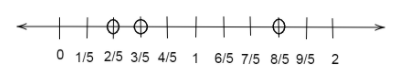Draw a number line and locate points on the line.$\dfrac{2}{5},\dfrac{3}{5},\dfrac{8}{5},\dfrac{4}{5}$Verified
147.3k+ views
Hint: Here we look at the denominator of each number and figure out how many parts we need to divide the number line, and using the concept of fractions we plot these points on the number line.

We are given four points $\dfrac{2}{5},\dfrac{3}{5},\dfrac{8}{5},\dfrac{4}{5}$ to be drawn on the number line.
Looking at the denominator of each fraction we can tell that the number of parts in which each part of the number line between two integers is divided. Here the number in the denominator is 5. So, between every integer there are five parts.
Also, we calculate each value in decimal form which helps us to determine the range of the number line to be drawn.
Calculate the value of $\dfrac{2}{5},\dfrac{3}{5},\dfrac{8}{5},\dfrac{4}{5}$.
$\dfrac{2}{5} = 0.4 \\ \dfrac{3}{5} = 0.6 \\ \dfrac{8}{5} = 1.6 \\ \dfrac{4}{5} = 0.8 \\$
Since the values in ascending order are $0.4,0.6,0.8,1.6$.We can see the range to be drawn on the number line is from 0 to 2.
We will divide the area between each integer in five parts and name the dividing line as the fraction.
So, the first dividing line will be $\dfrac{1}{5}$, second will be $\dfrac{2}{5}$ and so on then the fifth dividing line will be $\dfrac{5}{5} = 1$ which will become the integer one itself.
All values on the number line that are integers are always the fifth division line.Thus, the representation on the number line is as above.

Note: Students might make the mistake of representing $\dfrac{8}{5}$ in a wrong way as they think that all fractions with denominator 5 will be between 0 and 1. But we should always first check the value of the fraction and then plot it on the line.